Some Monohedral Tilings Derived From Regular Polygons

Paul Gailiunas

25 Hedley Terrace, Gosforth

Newcastle, NE3 1DP, England

email: Paul Gailiunas

Abstract

Some tiles derived from regular polygons can produce spiral tilings of the plane . This paper considers some more general classes of tilings with tiles derived from regular polygons, some have central symmetry, many have periodic symmetry, some have both, and a few have no symmetry at all. Any of these tiling patterns could be the basis for some interesting mathematical art, for example by colouring or decorating the tiles.

Using Regular Polygons to Make Tiles

Overlapping copies of a regular polygon around a common vertex produces a pattern of rhombi. A tile can be chosen bounded by sections of the perimeters of three of the polygons (figure 1). A section of the perimeter of a polygon will be termed a polygonal arc.

If the polygon is a regular n-gon then the angles of the rhombi are 1/n, 2/n, 3/n ... (measured as fractions of a full rotation; multiply by 360 for degrees, or by 2π for radians). If the arcs are measured in units equal to a side of a polygon then by inspection: t = r + s, Ð R = r/n, Ð S = (t - 1)/n, Ð T = 1/2 - s/nFigure 1. The construction of a tile.

Copies of any tile with r = 2 (or 1) will tile the plane. There are five types of isohedral tiling (every tile can be transformed into any other by a symmetry of the tiling, so all tiles are the same).identicallyreflectedrotated by180°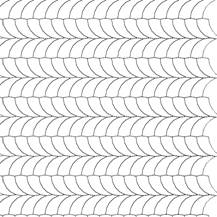reflected and rotated by180°

or the tiles are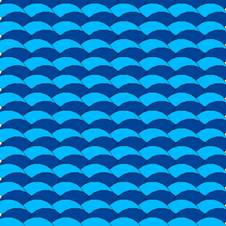not lying in visually obvious rows

The tiles meet at two types of vertex, where the angle sum = 1, for any value of n.

Figure 2. The tiling has only two types of vertex.

1/2 - 1/n + (t - 1)/n + 1/2 - s/n  =  1 + (t - s - 2)/n  =  1 + (r - 2)/n   =  1

2 (1/2 - 1/n) + r/n  =  1 - 2/n + r/n = 1

In general it will not be possible to tile the plane with tiles made like this because every convex section must be matched by a concave section, which can never occur. There are two special cases: if r = 1 then the arc is neither concave nor convex; if r = 2 then in all cases the tiles will fit as illustrated.

If ÐS is an exact fraction of a full rotation then a centrally symmetric tiling is possible.The tiling consists of V-shaped sections with ÐS at the apex.  Each section is in two parts. The tiles in one part are mirror images of the tiles in the other part. This is the underlying structure of all centrally-symmetric tilings with tiles derived from overlapping polygons.

There are some special tiles with unique properties. One, found by Robert Reid, has n = 12 and s = 2, so t = 4, since r must be 2. It forms a standard centrally symmetric tiling,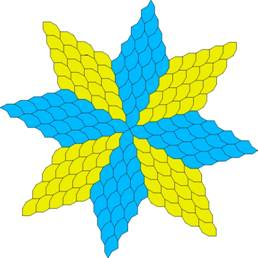and anotherthat is arranged around a dodecagon consisting of three tiles.  Another has n = 10 and s = 3, so t = 5 and Ð S = 2/5, the interior angle of a 10-gon, so the arcs r and t form a single arc of 7 sides. This tile has been investigated by Haresh Lalvani , and it will appear again as a special case of another type of tile. It allows spiral tilings of the plane .Biconcave Tiles

In figure 1, if the arc, r, is reflected in the line ST a new tile is produced. This is equivalent to removing a section from the tile that is bounded by two polygonal arcs that has apical angles of (r - 1)/n. The new tile has angles:

Ð R = r/n

Ð S = (t - 1)/n - (r - 1)/n

= (t - r)/n = s/n

Ð T = 1/2 - s/n - (r - 1)/n

= 1/2 - (r + s - 1) n = 1/2 - (t - 1)/n

This time the length of the convex arc is equal to the combined length of the concave arcs, and all such tiles will tile the plane. Figure 3 shows how three isohedral tilings are possible.

We can see that any of these tiles will work because for any tile the angle sum at each vertex of the tiling is always 1. The tile can be seen as a modified parallelogram.

Ð R + Ð S + Ð T + 1/2 - 1/n = r/n + s/n + 1/2 - (t - 1)/n + 1/2 - 1/n = 1 + (r + s - t)/n = 1Figure 3. All biconcave tiles will tile the plane.

When s = 2 there are two further possibilities because the tiles can fit together in a staggered fashion, related by either a rotation of 180°or a glide reflection.Robert Reid has discovered another possibility. If tiles can be arranged so that they meet at edges on convex polygonal arcs in principle tilings are possible with t = r + s + 2 (as in figure 2), but it works only if t = n/2 + 1. Again three isohedral tilings are possible: with only translational symmetry;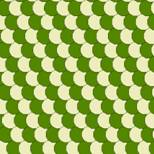with glide reflection;with glide reflection in another direction.Lalvani’s tile with n = 10, r = 2, s = 3, t = 5 can also be seen as an example of this type with n = 10, r = 1, s = 3, t = 6, since a polygonal arc of length 1 can be considered as either convex or concave.

If Ð R is an exact fraction of a full rotation it is possible to produce a centrally symmetric tiling that consists of two-part V-shaped sections in a similar way to the example in figure 3. When both r and s divide n two centrally symmetric tilings are possible, for example a tile with n = 18, r = 2, s = 3 will allow a tiling with rotational symmetry of order 6and another of order 9.Similarly the previous example of one of Reid’s tiles with n = 12, r = 3, s = 4 will allow a tiling with rotational symmetry of order 3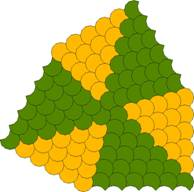and another of order 4.The value of t is determined by r and s, and four tiles will always fit around a vertex if angles of each type are present (as in figure 3), so all such tilings with the same values of r/n and s/n have identical structures. In the limit the tiles will have sides that are circular arcs (provided that they have the same radius, and the arc lengths satisfy t = r + s). Provided that it does not depend on some specific number (such as the tilings like figure 2, when r must be 2) any tiling has an infinite series of equivalent tilings, including the limiting one with circular arcs. The general case of spiral tilings described in  is of this type, although the special cases (most of the examples) are not, since they depend on particular number properties.

An interesting example is provided by the three-armed spiral using a tile from a hexagon (figure 13 in ). Doubling the values of n, r and s gives a tile with n = 12, r = 2, s = 4, t = 6 with the special property that it can be dissected into two tiles with n = 12, r = 1, s = 4, t = 5. Two three-armed spirals that use this tile were described in , this is a third (figure 4). Figure 5 shows the limiting case of this tiling, when the edges of the tiles are circular arcs.Figure 4. A three-armed spiral tiling dissected.Figure 5. A three-armed spiral tiling with circular arcs.

Special Cases

Figure 6 shows an additional way that some tiles can fit together. Usually there are no further tilings, apart from the cases when s = 1 and s = 2.

There are two types of vertex, both valency = 3. The same condition is satisfied by both types

Ð R + Ð S = 1/2 - 1/n

r/n + s/n = t/n = 1/2 - 1/n

t = n/2 1

Ð T = 2/n

1/2 - (t - 1)/n = 2/n

t = n/2 1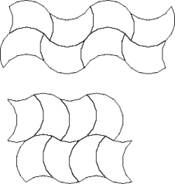Figure 6. An additional type of tiling.

If s = 2 the tiles can also fit in a staggered way that is analogous to the tiling in figure 2. The tile with n = 8, r = 1, s = 2 (so that t = 3 = n/2 ‑ 1) is special in several ways, and allows eleven different isohedral tilings, five as described in the first section since the side of l can be considered to be convex (Grünbaum and Shephard types 41,47,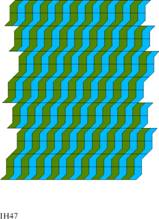43,42,44),two of the staggered type since s = 2 (types 2,4),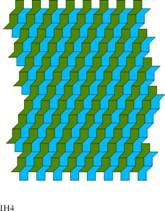one of the special type since t  = n/2 – 1 (type 6),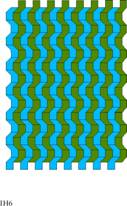one that is peculiar to tiles with r = 1 (type 53),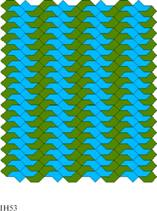and two that are possible because the tile with n = 8, r = 2, s = 2 can be dissected into two copies of it (types 43,51).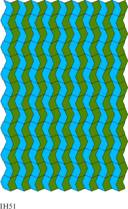It is quite easy to produce a tile that will allow any number of different isohedral tilings . This tile allows ten different types of isohedral tiling, which is probably the greatest number of any tile.

The tile with n = 10, r = 2, s = 2 (so that t = 4 = n/2 ‑ 1) is even more remarkable. It has mirror symmetry, so there are fewer different isohedral tilings, but it is possible to form boundaries in quite flexible ways between areas of isohedral tilingthat are oriented in different directions. Since s = 2 the tiles can also fit in a staggered way, allowing boundaries to meet, either symmetrically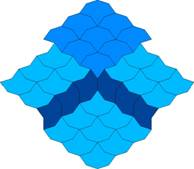or asymmetrically.A centrally symmetric tilingcan be constructed using this feature. A particularly unusual tiling has an additional rowinserted, breaking the symmetry.

Another centrally symmetric tiling can be seen as a spiral of either sense.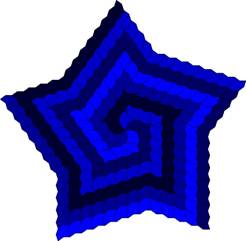Periodic Tilings

There are two cases when central regions of centrally-symmetric tilings will themselves tile the plane periodically: when they are hexagonal or square, possibly modified. Figure 7 illustrates how the tile with n = 6, r = 1, s = 2, t = 3 can form hexagons of various sizes, but there are equivalent tilings with these values multiplied by any integer. As we have already seen (figure 4) the special case when they are doubled produces a tile that can be dissected into two smaller congruent tiles (n = 12, r = 1, s = 4, t = 5). The resulting tilings, based on either basic hexagonsor the enlarged version can be quite attractive.The process of enlargement can continued indefinitely.Figure 7. Periodic tilings based on hexagons.

The square is the basis for the other type of periodic tiling (n = 4, r = 1, s = 1, t = 2), and again the case when these values are doubled is special because it produces a tile that can be dissected into two smaller congruent tiles (as in isohedral tilings type 43and 51above), but in two different ways, since it has mirror symmetry (figure 8). This allows many variations of the basic tiling.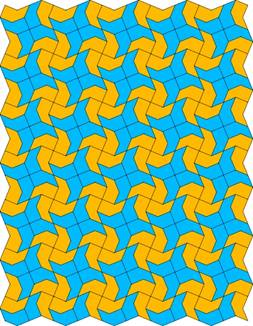Again enlargement is possible,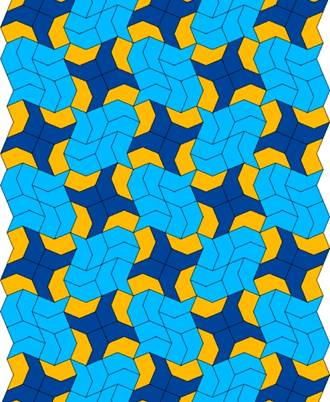with different dissections,and the enlargement can be continued.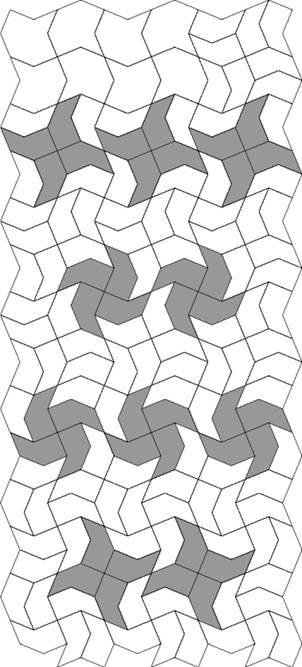Figure 8. Basic variations on the periodic tiling based on squares.

Further Possibilities

Although a few tilings with tiles having r = 1 have been considered there are probably many more to be found, since the constraint imposed by matching concave and convex arcs is relaxed.  There has been no consideration of tilings derived from polygons having an odd number of sides, which seem to be more limited, but they are known to allow at least one type of spiral tiling , and many tilings using the reflex equilateral pentagon are known .  Versatiles  appear in a range of spiral tilings, but only the pentagonal versatile  seems to allow a particularly wide range of tilings, nevertheless there are probably more to be discovered. Another possibility yet to be explored in any detail is the use tiles with boundaries consisting of more than three polygonal arcs.

Many of these tilings are aesthetically pleasing as they are, and they can be coloured in many different ways. The resulting patterns could have applications in architectural details (literally tilings), or in textile design, or simply as works of mathematical art in their own right.

References

 Gailiunas P., "Spiral Tilings" Bridges 2000, pp.133-140. Copied at http://www.mi.sanu.ac.yu/vismath/gal/index.html

 US Patent 4,620,998,  1986, cited in Lalvani H., Meta Archtecture, in Architecture and Science (ed. Di Cristina G.), Wiley Academy, 2001.

 Stock D.L. and Wichmann B.A., "Odd Spiral Tilings", Mathematics Magazine vol.73, no.5, Dec 2000, pp.339-347.

 Grünbaum B. and Shephard G.C., Tilings and Patterns, Freeman, 1987, p.50.

 Hatch G. "Tessellations with Equilateral Reflex Polygons", Mathematics Teaching, no.84, Sept.1978, p.32.

 Simonds D.R., "Central Tessellations with an Equilateral Pentagon", Mathematics Teaching, no.81, Dec. 1977, p.36.

 Grünbaum B. and Shephard G.C., "Spiral Tilings and Versatiles", Mathematics Teaching, no.88, Sept. 1979, pp.50‑1.

 Rice M. and Schattschneider D., "The Incredible Pentagonal Versatile", Mathematics Teaching, no.93, Dec. 1980, pp.52‑3.